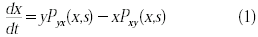## Main

Previous models of language dynamics have focused on the transmission and evolution of syntax, grammar or other structural properties of a language itself2,3,4,5,6,7. In contrast, the model we describe here idealizes languages as fixed, and as competing with each other for speakers. For simplicity, we also assume a highly connected population, with no spatial or social structure, in which all speakers are monolingual.

Consider a system of two competing languages, X and Y, in which the attractiveness of a language increases with both its number of speakers and its perceived status8 (a parameter that reflects the social or economic opportunities afforded to its speakers). Suppose an individual converts from Y to X with a probability, per unit of time, of Pyx(x,s), where x is the fraction of the population speaking X, and 0≤s≤1 is a measure of X's relative status. A minimal model for language change is thereforewhere y = 1 − x is the complementary fraction of the population speaking Y at time t. By symmetry, interchanging languages should yield the same transition probability as a swap in the fraction of speakers and relative status; thus Pxy(x,s) = Pyx(1 − x,1 − s). We also assume that no one will adopt a language that has no speakers (Pyx(0,s) = 0) or no status (Pyx(x,0) = 0), and that Pyx is smooth and monotonically increasing in both arguments.

These mild assumptions imply that equation (1) generically has three fixed points. Of these, only x = 0 and x = 1 are stable. The model therefore predicts that two languages cannot coexist stably — one will eventually drive the other to extinction.

To test our model, we collected data on the number of speakers of endangered languages in 42 regions of Peru, Scotland, Wales, Bolivia, Ireland and Alsace-Lorraine, four instances of which are shown in Fig. 1. We fit the model's solutions to the data, assuming transition functions of the forms Pyx(x,s) = cxas and Pxy(x,s) = c(1 − x)a(1 − s). Unexpectedly, the exponent a was found to be roughly constant across cultures, with a = 1.31 ± 0.25 (mean ± standard deviation; further details are available from the authors).